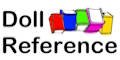Doll Auctions | Doll Books | Dolls For Sale | Forum | Doll Glossary | Doll Marks | Doll Values

Doll Categories

Vintage Ideal Toni Doll and Clothes 1949-1953

 By Country American | English By Material  By Type

related  Ideal Dolls 1906-1939 | 1940s | 1950s | 1960s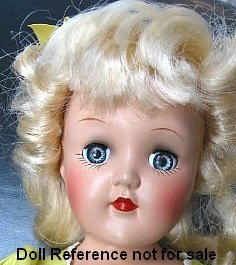Ideal Toni doll, 14" tall doll marked P-90 The Ideal Toni doll was introduced in 1949 as an advertising doll for Toni Home Permanent.  Toni is made of all hard plastic, has a jointed body, the earliest dolls wore a nylon wig, she has sleep eyes usually in blue but other colors too, real upper eyelashes, painted lower lashes, a small rosebud painted closed mouth and was a loved doll by many little girls and today by doll collectors of all ages. Toni dolls are almost always found marked as follows: P-90 = 14", P-91 = 16", P-92 = 19", P-93 = 21", P-94 = 23" tall

Ideal Toni Dolls and Clothes Identified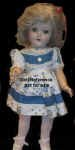Toni P-90 = 14"Toni P-90 = 14"Toni P-90 = 14"Toni P-90 = 14"Toni P-90 = 14" Toni P-90 = 14"Toni P-90 = 14"Toni P-90 = 14"Toni P-90 = 14"Toni P-90 = 14"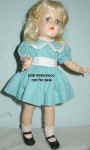Toni P-90 = 14"Toni P-90 = 14"Toni P-90 = 14"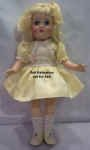Toni P-90 = 14"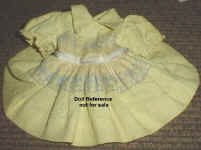Toni P-90 = 14"Toni P-90 = 14" Toni P-90 = 14" Toni P-90 = 14" Ideal's Toni P-91 is 16" tall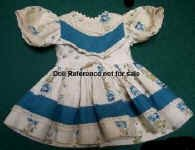Toni P-91 = 16"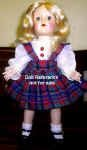Toni P-91 = 16"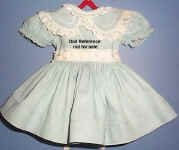Toni P-91 = 16"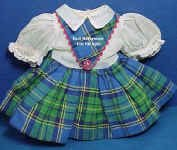Toni P-91 = 16"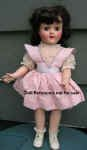Toni P-91 = 16"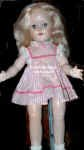Toni P-91 = 16" Toni P-91 = 16"Toni P-91 = 16"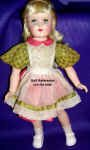Toni P-93 = 21"Toni P-93 = 21"Toni ? size - dress is shown from the back

related  Ideal Dolls 1906-1939 | 1940s | 1950s | 1960s

All trademarks are respective of their owners and have no affiliation to this website